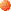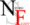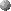Neuro-Fuzzy SystemsNeuro-Fuzzy Software# What are Neuro-Fuzzy Systems?

A neuro-fuzzy system is a fuzzy system that uses a learning algorithm derived from or inspired by neural network theory to determine its parameters (fuzzy sets and fuzzy rules) by processing data samples.

This is the abstract of our view on neuro-fuzzy systems which we explain in more detail below.Modern neuro-fuzzy systems are usually represented as special multilayer feedforward neural networks (see for example models like ANFIS , FuNe , Fuzzy RuleNet , GARIC , or NEFCLASS and NEFCON ). However, fuzzifications of other neural network architectures are also considered, for example self--organizing feature maps [2, 10]. In those neuro--fuzzy networks, connection weights and propagation and activation functions differ from common neural networks. Although there are a lot of different approaches [3, 4, 7, 8], we usually use the term neuro--fuzzy system for approaches which display the following properties:A neuro-fuzzy system is based on a fuzzy system which is trained by a learning algorithm derived from neural network theory. The (heuristical) learning procedure operates on local information, and causes only local modifications in the underlying fuzzy system.A neuro-fuzzy system can be viewed as a 3-layer feedforward neural network. The first layer represents input variables, the middle (hidden) layer represents fuzzy rules and the third layer represents output variables. Fuzzy sets are encoded as (fuzzy) connection weights. It is not necessary to represent a fuzzy system like this to apply a learning algorithm to it. However, it can be convenient, because it represents the data flow of input processing and learning within the model. Remark: Sometimes a 5-layer architecture is used, where the fuzzy sets are represented in the units of the second and fourth layer.A neuro-fuzzy system can be always (i.e.\ before, during and after learning) interpreted as a system of fuzzy rules. It is also possible to create the system out of training data from scratch, as it is possible to initialize it by prior knowledge in form of fuzzy rules. Remark: Not all neuro-fuzzy models specifiy learning procedures for fuzzy rule creation.The learning procedure of a neuro-fuzzy system takes the semantical properties of the underlying fuzzy system into account. This results in constraints on the possible modifications applicable to the system parameters. Remark: Not all neuro-fuzzy approaches have this property.A neuro-fuzzy system approximates an \$n\$-dimensional (unknown) function that is partially defined by the training data. The fuzzy rules encoded within the system represent vague samples, and can be viewed as prototypes of the training data. A neuro-fuzzy system should not be seen as a kind of (fuzzy) expert system, and it has nothing to do with fuzzy logic in the narrow sense.## References

1. H. R. Berenji and P. Khedkar, Learning and tuning fuzzy logic controllers through reinforcements. IEEE Trans. Neural Networks 3 (1992) 724--740.
2. J. C. Bezdek, E. C.-K. Tsao, and N. R. Pal, Fuzzy Kohonen clustering networks, in: Proc. IEEE Int. Conf. on Fuzzy Systems (San Diego, 1992) 1035--1043.
3. J. J. Buckley and Y. Hayashi, Fuzzy neural networks: A survey, Fuzzy Sets and Systems 66 (1994) 1-13.
4. J. J. Buckley and Y. Hayashi, Neural networks for fuzzy systems, Fuzzy Sets and Systems 71 (1995) 265-276.
5. S. K. Halgamuge and M. Glesner, Neural networks in designing fuzzy systems for real world applications, Fuzzy Sets and Systems 65 (1994) 1--12.
6. J.-S.R. Jang, ANFIS: Adaptive-Network-Based Fuzzy Inference Systems, IEEE Trans. Systems, Man & Cybernetics 23 (1993) 665--685
7. D. Nauck, F. Klawonn, and R. Kruse, Foundations of Neuro--Fuzzy Systems, (Wiley, Chichester, 1996, to be published).
8. D. Nauck and R. Kruse, Designing neuro--fuzzy systems through backpropagation, in: W. Pedrycz, Ed., Fuzzy Modelling: Paradigms and Practice, (Kluwer, Boston, 1996) 203-228.
9. N. Tschichold-Gürman, RuleNet -- A New Knowledge--Based Artificial Neural Network Model with Application Examples in Robotics, PhD thesis (ETH Zürich, 1996).
10. P. Vuorimaa, Fuzzy self--organizing map, Fuzzy Sets and Systems, 66 (1994) 223--231.See also our list of online papers, and our list of publications.Detlef Nauck (nauck@iik.cs.uni-magdeburg.de), Feb. 7, 1997 18:35